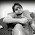Only Engineers Can Solve This Puzzle. Try if you can.

1 + 4  = 5
2 + 5 = 12
3 + 6  = 21
5 + 8 = ??

SHARE IF YOU GOT IT

Solving math puzzles help you in exams and interviews. Follow this website to get more puzzles

44
(5 x 8) + 4

1.How to get 44, 1+4=5 4×1=4+1=5,2+5=12 2×5=10+2=12,3+6=21 3×6=18+3=21,missing 4+7=32 7×4=28+4=32, 8+5=45 8×5=40+5=45

2.45

3.45
4.5.6.7.8.